Area Of Sphere

Chapter 13 Class 9 Surface Areas and Volumes
Concept wise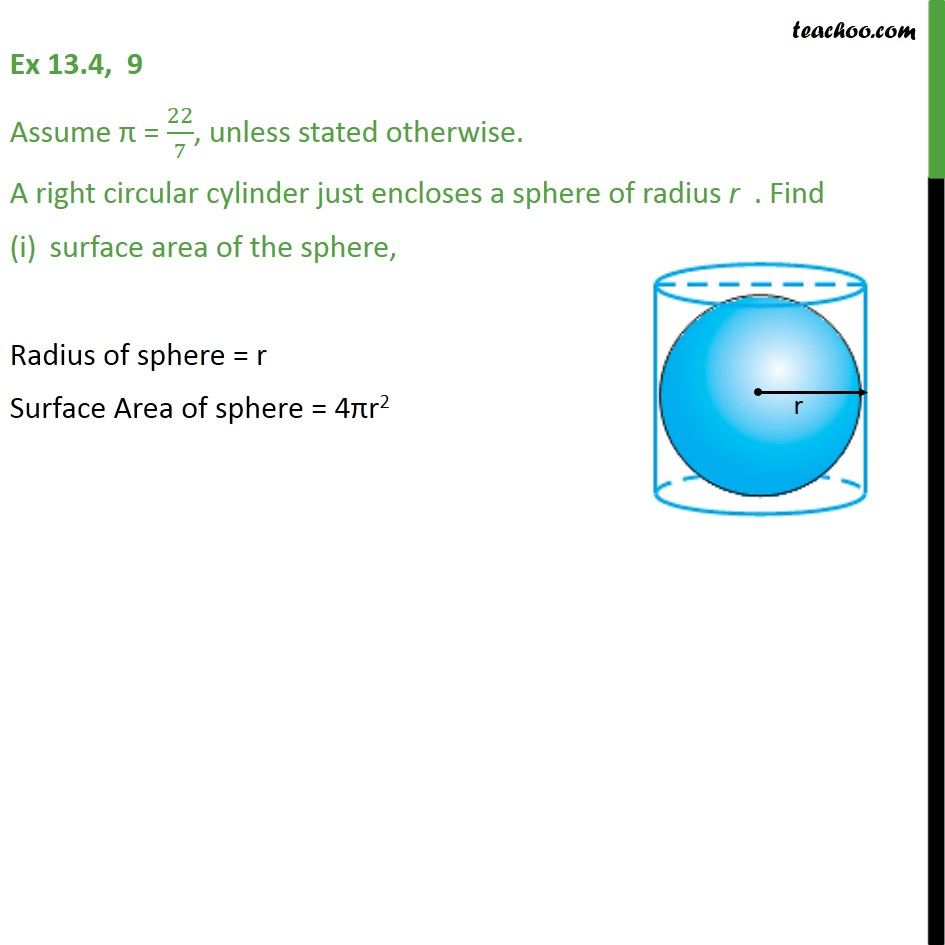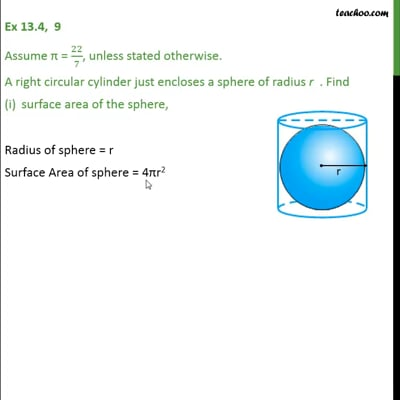This video is only available for Teachoo black users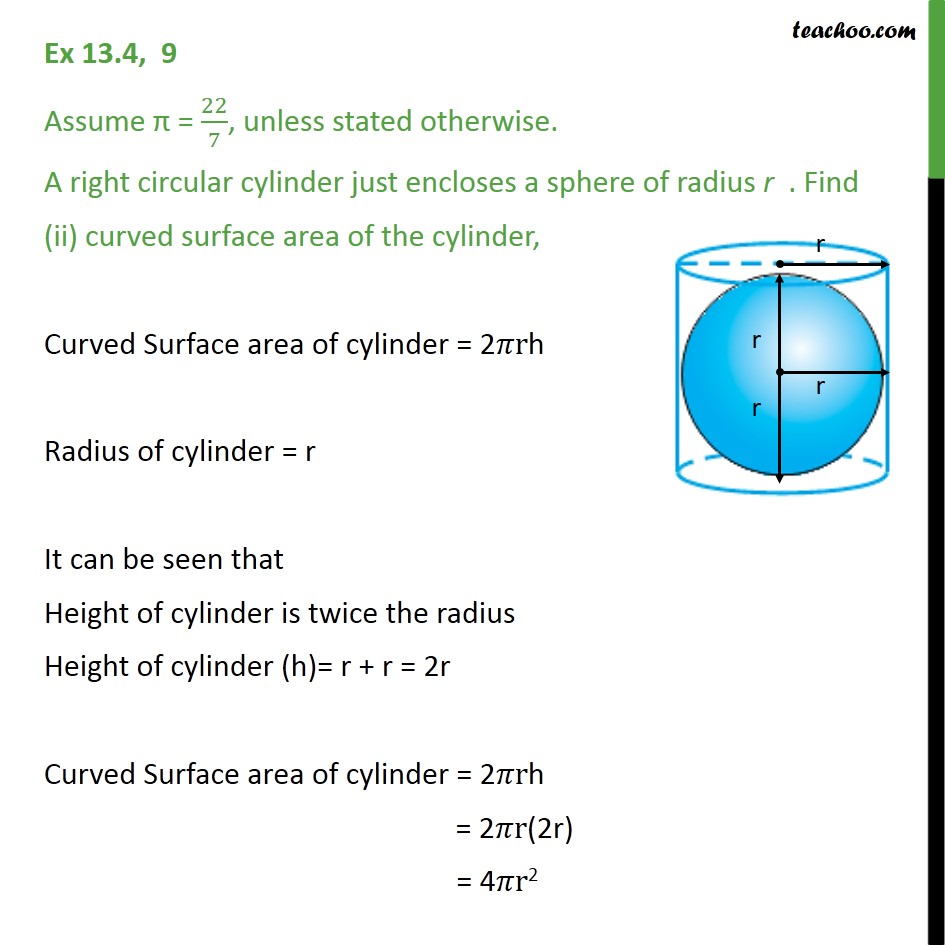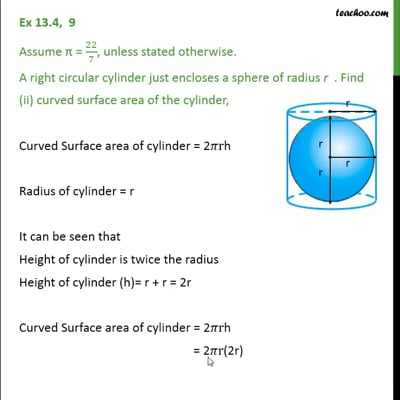This video is only available for Teachoo black users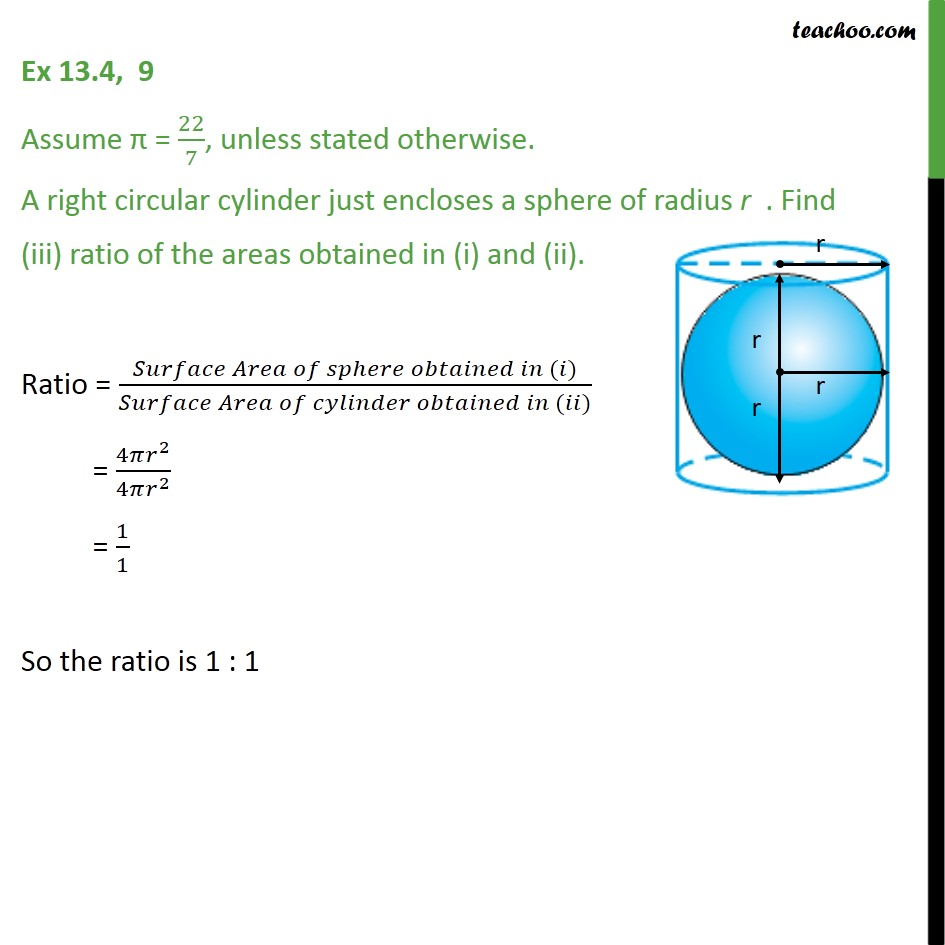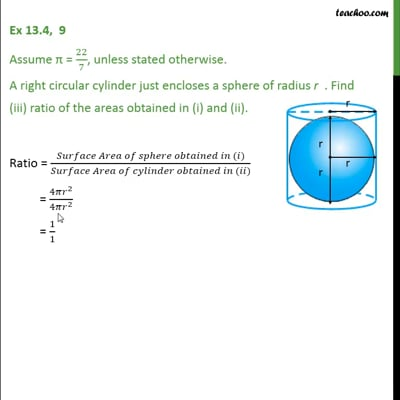This video is only available for Teachoo black users

Introducing your new favourite teacher - Teachoo Black, at only ₹83 per month

### Transcript

Ex 13.4, 9 Assume π = 22/7, unless stated otherwise. A right circular cylinder just encloses a sphere of radius r . Find surface area of the sphere, Radius of sphere = r Surface Area of sphere = 4πr2 Ex 13.4, 9 Assume π = 22/7, unless stated otherwise. A right circular cylinder just encloses a sphere of radius r . Find (ii) curved surface area of the cylinder, Curved Surface area of cylinder = 2𝜋rh Radius of cylinder = r It can be seen that Height of cylinder is twice the radius Height of cylinder (h)= r + r = 2r Curved Surface area of cylinder = 2𝜋rh = 2𝜋r(2r) = 4𝜋r2 Ex 13.4, 9 Assume π = 22/7, unless stated otherwise. A right circular cylinder just encloses a sphere of radius r . Find (iii) ratio of the areas obtained in (i) and (ii). Ratio = (𝑆𝑢𝑟𝑓𝑎𝑐𝑒 𝐴𝑟𝑒𝑎 𝑜𝑓 𝑠𝑝ℎ𝑒𝑟𝑒 𝑜𝑏𝑡𝑎𝑖𝑛𝑒𝑑 𝑖𝑛 (𝑖))/(𝑆𝑢𝑟𝑓𝑎𝑐𝑒 𝐴𝑟𝑒𝑎 𝑜𝑓 𝑐𝑦𝑙𝑖𝑛𝑑𝑒𝑟 𝑜𝑏𝑡𝑎𝑖𝑛𝑒𝑑 𝑖𝑛 (𝑖𝑖)) = (4𝜋𝑟^2)/(4𝜋𝑟^2 ) = 1/1 So the ratio is 1 : 1